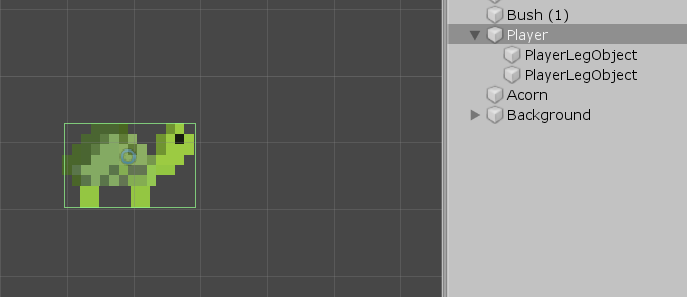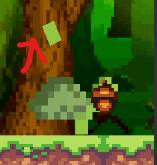# Rotation of gameobject to a given angle?

Novice question here. I’m having difficulty coding the rotation of my character’s legs back to a standing position after he’s stopped moving. Essentially, I made the legs of the character as children of the parent character model, because I wanted them to sort of rotate freely without needing to animate them under the influence of physics. I realized that since my game is 2D and I locked the actual character’s rotation, it means that the legs won’t be able to rotate either, and I have to script it manually. I’ve gotten it to go back to its original position from where it ends up after moving (I’m just adding torque right now to cause some rotation, but not randomly varying the torque over time, which I plan on implementing), but it seems inconsistent and will go back sometimes but not others, or do it slowly. Also, if I jump, the leg I applied the script to doesn’t seem to follow the parent’s y movement, and it becomes disconnected from my character’s body. I know I could probably do this more easily through animation, but I’ve consistently had trouble with various rotation implementations (like quaternions and lerping and whatnot) in the past, and I want to improve that and see if I can understand why I’m getting this behavior. Here’s a sample of the script I’m using to move the legs:

``````public class LegMovement : MonoBehaviour
{
//init
Rigidbody2D rb2d;
PlayerController2D player;
ConstantForce2D force2D;
private bool rotatingToZero = false; // used to flag when the player has stopped moving and the legs should rotate back to their default positions
// Start is called before the first frame update
void OnEnable()
{
rb2d = GetComponent<Rigidbody2D>();
player = FindObjectOfType<PlayerController2D>();
}
// Update is called once per frame
void Update()
{
if(player.isMoving == true)
{
rotatingToZero = false;
}
else
{
rotatingToZero = true;
Vector3 to = new Vector3(0, 0, 0); // going to be rotating to a local angle of 0 degrees
if (Vector3.Distance(transform.eulerAngles, to) > 0.01f) //if the distance in degrees from the current angle to the desired angle is greater than 0.01 (magnitude, so won't be negative)
{
transform.eulerAngles = Vector3.Lerp(transform.rotation.eulerAngles, to, Time.deltaTime*3.0f);
}
else //this is for when it's within 0.01 distance, because the Lerp will go on forever
{
transform.eulerAngles = to;
rotatingToZero = false;
}
//if (rb2d.rotation < 0)
// {
//while(rb2d.rotation != 0)
/*for (float i = rb2d.rotation; i < 0; i += 0.001f)
{
if (rb2d.rotation == 0)
{
return;
}
}*/
/* float originalAngle = transform.rotation.eulerAngles.y;
float zeroAngle = 0f;
transform.rotation = Quaternion.AngleAxis(originalAngle + (Time.deltaTime * 10f), Vector3.up);
rb2d.rotation += Time.deltaTime * 10f;
*/

//}
//else if (rb2d.rotation > 0)
//{
//while(rb2d.rotation != 0)
/*for (float i = rb2d.rotation; i > 0; i -= 0.001f)
{

if (rb2d.rotation == 0)
{
return;
}
}*/
/*float originalAngle = transform.rotation.y;
float zeroAngle = 0f;
transform.rotation = Quaternion.AngleAxis(originalAngle + (Time.deltaTime * -10f), Vector3.up);
rb2d.rotation += Time.deltaTime * -10f;*/
//}
Debug.Log(rb2d.angularVelocity);
}
}
}
``````

Here’s a picture of how I have the character set up:And here’s a picture of what happens if I have the character jump and the leg I attached the script to doesn’t follow; the green rectangle that the red arrow is pointing to is the leg (other leg is only fine because I didn’t test the script on it yet):